Next: About this document Up: My Home Page

# Hashing Lecture 21

Steven S. Skiena

Hashing

One way to convert form names to integers is to use the letters to form a base ``alphabet-size'' number system:

To convert ``STEVE'' to a number, observe that e is the 5th letter of the alphabet, s is the 19th letter, t is the 20th letter, and v is the 22nd letter.

Thus ``Steve''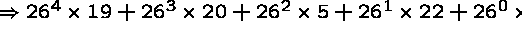Thus one way we could represent a table of names would be to set aside an array big enough to contain one element for each possible string of letters, then store data in the elements corresponding to real people. By computing this function, it tells us where the person's phone number is immediately!!

What's the Problem?

Because we must leave room for every possible string, this method will use an incredible amount of memory. We need a data structure to represent a sparse table, one where almost all entries will be empty.

We can reduce the number of boxes we need if we are willing to put more than one thing in the same box!

Example: suppose we use the base alphabet number system, then take the remainder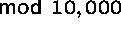Now the table is much smaller, but we need a way to deal with the fact that more than one, (but hopefully every few) keys can get mapped to the same array element.

The Basics of Hashing

The basics of hashing is to apply a function to the search key so we can determine where the item is without looking at the other items. To make the table of reasonable size, we must allow for collisions, two distinct keys mapped to the same location.

• We a special hash function to map keys (hopefully uniformly) to integers in a certain range.
• We set up an array as big as this range, and use the valve of the function as the index to store the appropriate key. Special care must be taken to handle collisions when they occur.

There are several clever techniques we will see to develop good hash functions and deal with the problems of duplicates.

Hash Functions

The verb ``hash'' means ``to mix up'', and so we seek a function to mix up keys as well as possible.

The best possible hash function would hash m keys into n ``buckets'' with no more than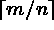keys per bucket. Such a function is called a perfect hash function

How can we build a hash function?

Let us consider hashing character strings to integers. The ORD function returns the character code associated with a given character. By using the ``base character size'' number system, we can map each string to an integer.

The First Three SSN digits Hash

The first three digits of the Social Security Number

The last three digits of the Social Security Number

What is the big picture?

1. A hash function which maps an arbitrary key to an integer turns searching into array access, hence O(1).
2. To use a finite sized array means two different keys will be mapped to the same place. Thus we must have some way to handle collisions.
3. A good hash function must spread the keys uniformly, or else we have a linear search.

Ideas for Hash Functions

• Truncation - When grades are posted, the last four digits of your SSN are used, because they distribute students more uniformly than the first four digits.
• Folding - We should get a better spread by factoring in the entire key. Maybe subtract the last four digits from the first five digits of the SSN, and take the absolute value?
• Modular Arithmetic - When constructing pseudorandom numbers, a good trick for uniform distribution was to take a big number mod the size of our range. Because of our roulette wheel analogy, the numbers tend to get spread well if the tablesize is selected carefully.

Prime Numbers are Good Things

Suppose we wanted to hash check totals by the dollar value in pennies mod 1000. What happens?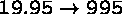,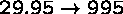, and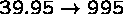Prices tend to be clumped by similar last digits, so we get clustering.

If we instead use a prime numbered Modulus like 1007, these clusters will get broken: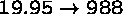,, and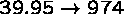.

In general, it is a good idea to use prime modulus for hash table size, since it is less likely the data will be multiples of large primes as opposed to small primes - all multiples of 4 get mapped to even numbers in an even sized hash table!

The Birthday Paradox

No matter how good our hash function is, we had better be prepared for collisions, because of the birthday paradox.

Assuming 365 days a year, what is the probability that exactly two people share a birthday? Once the first person has fixed their birthday, the second person has 365 possible days to be born to avoid a collision, or a 365/365 chance.

With three people, the probability that no two share is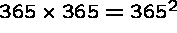. In general, the probability of there being no collisions after n insertions into an m-element table is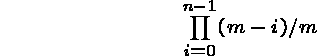When m = 366, this probability sinks below 1/2 when N = 23 and to almost 0 when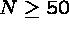.

The moral is that collisions are common, even with good hash functions.

What about Collisions?

No matter how good our hash functions are, we must deal with collisions. What do we do when the spot in the table we need is occupied?

• Put it somewhere else! - In open addressing, we have a rule to decide where to put it if the space is already occupied.
• Keep a list at each bin! - At each spot in the hash table, keep a linked list of keys sharing this hash value, and do a sequential search to find the one we need. This method is called chaining.

Collision Resolution by Chaining

The easiest approach is to let each element in the hash table be a pointer to a list of keys.

Insertion, deletion, and query reduce to the problem in linked lists. If the n keys are distributed uniformly in a table of size m/n, each operation takes O(m/n) time.

Chaining is easy, but devotes a considerable amount of memory to pointers, which could be used to make the table larger. Still, it is my preferred method.

Open Addressing

We can dispense with all these pointers by using an implicit reference derived from a simple function:

If the space we want to use is filled, we can examine the remaining locations:

1. Sequentially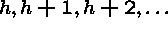2. Quadratically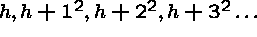3. Linearly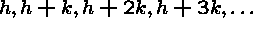The reason for using a more complicated scheme is to avoid long runs from similarly hashed keys.

Deletion in an open addressing scheme is ugly, since removing one element can break a chain of insertions, making some elements inaccessible.

Performance on Set Operations

With either chaining or open addressing:

• Search - O(1) expected, O(n) worst case.
• Insert - O(1) expected, O(n) worst case.
• Delete - O(1) expected, O(n) worst case.

Pragmatically, a hash table is often the best data structure to maintain a dictionary. However, the worst-case running time is unpredictable.

The best worst-case bounds on a dictionary come from balanced binary trees, such as red-black trees.Next: About this document Up: My Home Page

Steve Skiena
Mon Nov 10 15:33:24 EST 1997